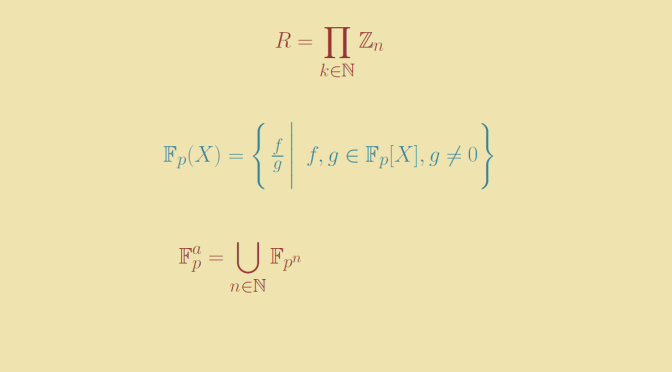Infinite rings and fields with positive characteristic

Familiar to us are infinite fields whose characteristic is equal to zero like $$\mathbb Z, \mathbb Q, \mathbb R$$ or the field of constructible numbers.

We’re also familiar with rings having infinite number of elements and zero for characteristic like:

• The rings of polynomials $$\mathbb Z[X], \mathbb Q[X], \mathbb R[X]$$.
• The rings of matrices $$\mathcal{M}_2(\mathbb R)$$.
• Or the ring of real continuous functions defined on $$\mathbb R$$.

We also know rings or fields like integers modulo $$n$$ (with $$n \ge 2$$) $$\mathbb Z_n$$ or the finite field $$\mathbb F_q$$ with $$q=p^r$$ elements where $$p$$ is a prime.

We provide below examples of infinite rings or fields with positive characteristic.

Infinite rings with positive characteristic

Consider the ring $$\mathbb Z_n[X]$$ of polynomials in one variable $$X$$ with coefficients in $$\mathbb Z_n$$ for $$n \ge 2$$ integer. It is an infinite ring since $$\mathbb X^m \in \mathbb{Z}_n[X]$$ for all positive integers $$m$$, and $$X^r \neq X^s$$ for $$r \neq s$$. But the characteristic of $$\mathbb Z_n[X]$$ is clearly $$n$$.

Another example is based on product of rings. If $$I$$ is an index set and $$(R_i)_{i \in I}$$ a family of rings, one can define the product ring $$\displaystyle \prod_{i \in I} R_i$$. The operations are defined the natural way with $$(a_i)_{i \in I} + (b_i)_{i \in I} = (a_i+b_i)_{i \in I}$$ and $$(a_i)_{i \in I} \cdot (b_i)_{i \in I} = (a_i \cdot b_i)_{i \in I}$$. Fixing $$n \ge 2$$ integer and taking $$I = \mathbb N$$, $$R_i = \mathbb Z_n$$ for all $$i \in I$$ we get the ring $$\displaystyle R = \prod_{k \in \mathbb N} \mathbb Z_n$$. $$R$$ multiplicative identity is the sequence with all terms equal to $$1$$. The characteristic of $$R$$ is $$n$$ and $$R$$ is obviously infinite.

Finally, consider and infinite set $$X$$ and consider the ring $$(R, \Delta, \cap)$$ where $$R=\mathcal{P}(X)$$ is the power set of $$X$$, $$\Delta$$ the symmetric difference (used as addition) and $$\cap$$ the intersection (used as multiplication). Once can prove that $$(\mathcal{P}(X), \Delta, \cap)$$ is a ring where $$X$$ is the multiplicative identity. The characteristic of $$R$$ is equal to $$2$$ as $$X \Delta X = \emptyset$$.

Infinite fields with positive characteristic

For our first example of an infinite field having a positive characteristic, we consider $\mathbb{F}_p(X)=\left\{\,\frac{f}{g}\,\Bigg|\,\,\,f,g\in\mathbb{F}_p[X], g\neq0\right\},$ the rational functions in the indeterminate $$X$$ with coefficients in $$\mathbb{F}_p$$ where $$p$$ is a prime. The field $$\mathbb{F}_p(X)$$ is infinite as it contains $$1, X, X^2, \dots$$ and it is of characteristic $$p$$ because it contains $$\mathbb{F}_p$$ (alternatively, because the kernel of the unique ring homomorphism $$\mathbb{Z} \to \mathbb{F}_p(X)$$ is $$p \mathbb{Z}$$).

Our second example is $\mathbb{F}_p^a=\bigcup_{n \in \mathbb N} \mathbb{F}_{p^n},$ the algebraic closure of $$\mathbb{F}_p$$. The main arguments to prove that $$\bigcup_{n \in \mathbb N} \mathbb{F}_{p^n}$$ is the algebraic closure of $$\mathbb{F}_p$$ are the following ones. Let $$q=p^n$$. Then $$\mathbb{F}_p^a$$ contains a unique splitting field of $$f(X) = X^q-X$$ over $$\mathbb{F}_p$$. Let $K = \{\alpha \in \mathbb{F}_p^a \, | \, f(\alpha)=0\}.$ Then $$K$$ is a field. The polynomial $$X^q-X$$ is separable since its derivative is equal to $$-1$$. Hence $$K$$ has $$q$$ elements. Therefore $$K$$ is the finite field with $$q$$ elements. Conversely, let $$a$$ be algebraic over $$\mathbb{F}_p$$ with $$[\mathbb{F}_p(a):\mathbb{F}_p]=n$$. Then $$\mathbb{F}_p(a)$$ has $$p^n$$ elements. Hence $$a \in \mathbb{F}_{p^n}$$.